# Blackbody radiation and the cosmic microwave background

• I
• PainterGuy
PainterGuy
Hi,

The following is my basic understanding of blackbody radiation spectrum. The important sections are in boldface.

Black-body radiation has a characteristic, continuous frequency spectrum that depends only on the body's temperature, called the Planck spectrum or Planck's law. The spectrum is peaked at a characteristic frequency that shifts to higher frequencies with increasing temperature, and at room temperature most of the emission is in the infrared region of the electromagnetic spectrum.
...
A black body at room temperature radiates only in the infrared spectrum and cannot be perceived by the human eye,  but some reptiles can sense... As the object becomes a little hotter (about 500°C, 900°F or 800K), the emission spectrum gets stronger and extends into the human visual range, and the object appears dull red. As its temperature increases further, it emits more and more orange, yellow, green, and blue light (and ultimately beyond violet, ultraviolet).

Question 1:
The quote above says that at room temperature (let's say 20 C or 293 K) the emission is in the infrared region. You can see in the figure below, lower curve, that at temperature of 37 C (little higher than room temperature), the curve extends well into longer wavelengths such as microwave and beyond. Is it really true that a blackbody at 37 C radiates microwaves and radio waves etc. as shown in the figure below? I don't think so. Then, why is the curve shown this way?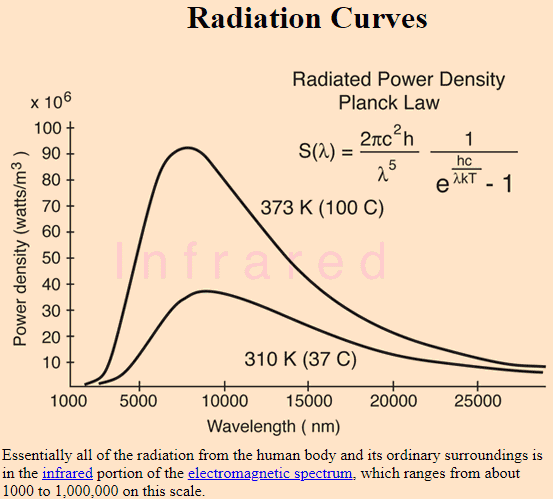Source: http://hyperphysics.phy-astr.gsu.edu/hbase/bbrc.html

Real objects never behave as full-ideal black bodies, and instead the emitted radiation at a given frequency is a fraction of what the ideal emission would be.

A black body radiates energy at all frequencies, but its intensity rapidly tends to zero at high frequencies (short wavelengths). For example, a black body at room temperature (300 K) with one square meter of surface area will emit a photon in the visible range (390–750 nm) at an average rate of one photon every 41 seconds, meaning that, for most practical purposes, such a black body does not emit in the visible range.

Question 2:
Most of the time I haven't seen that the size of blackbody is mentioned. For example, if sun is approximated as a blackbody, its temperature is almost 5800 K. There could be another blackbody of tennis ball size which is also at temperature of 5800 K. I don't think that power radiated by them is the same. The tennis ball size blackbody will radiate less power. The figure below uses spectral radiance units, kW/(sr.m^2.nm), but the figure above, used for Question 1, uses power density units, watts/m^3.

Which unit is more descriptive? Why size is not mentioned? Perhaps, the unit conveys this information indirectly.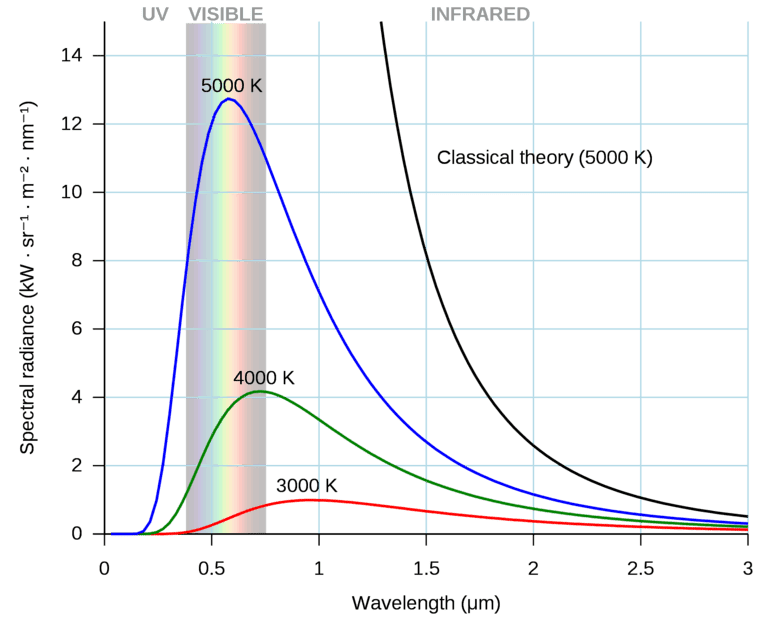Question 3:
The Wikipedia article, en.wikipedia.org/wiki/Black-body_radiation, says that an almost perfect black-body spectrum is exhibited by the cosmic microwave background radiation. It's related to Question 1.

Was CMB always more like a blackbody radiation, or it started acting more like radiation from a blackbody as the time passed and universe expanded?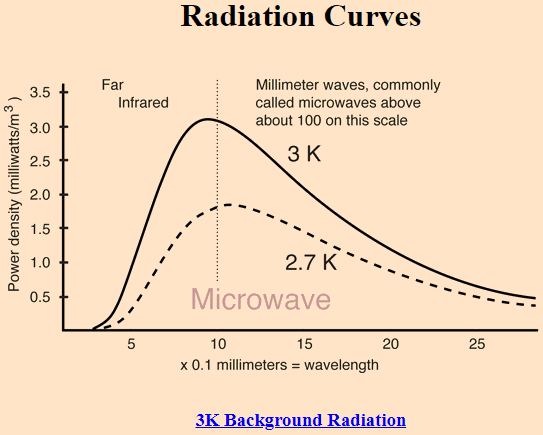Source: http://hyperphysics.phy-astr.gsu.edu/hbase/bbrc.html

Thank you for your help and time!

1: https://physics.stackexchange.com/questions/225207/is-sun-a-black-body
2: https://physics.stackexchange.com/q...-be-that-the-sun-emits-more-than-a-black-body

•pinball1970 and Delta2

Gold Member
I have my own way of explaining and visualizing this but a more technical treatment would @sophiecentaur 's bag

•sophiecentaur
Mentor
Is it really true that a blackbody at 37 C radiates microwaves and radio waves etc. as shown in the figure below? I don't think so.
Why are you skeptical? (I am not saying you are wrong, I just wonder about your thinking process here.)
Real objects never behave as full-ideal black bodies, and instead the emitted radiation at a given frequency is a fraction of what the ideal emission would be.
Correct. Non-metallic matter is mostly transparent to radio waves, so there would be no emission at long wavelengths.
I don't think that power radiated by them is the same. The tennis ball size blackbody will radiate less power.
For an ideal blackbody, the power emitted per unit area (of the surface) is the same. Of course, a larger object will emit more total power than a smaller one (c.f. the Stefan-Boltzmann law).
The figure below uses spectral radiance units, kW/(sr.m^2.nm), but the figure above, used for Question 1, uses power density units, watts/m^3.

Which unit is more descriptive? Why size is not mentioned? Perhaps, the unit conveys this information indirectly.
Spectral radiance is the direct result of the Planck distribution, which a distribution function and hence made to be integrated.
Was CMB always more like a blackbody radiation, or it started acting more like radiation from a blackbody as the time passed and universe expanded?
It was like that from the beginning. The only thing that can change is the wavelength of the individual photons (red-shift due to the expansion if the universe).

•PainterGuy, PeroK and Lord Jestocost
Gold Member
•PainterGuy
WernerQH
The figure below uses spectral radiance units, kW/(sr.m^2.nm), but the figure above, used for Question 1, uses power density units, watts/m^3.

Which unit is more descriptive? Why size is not mentioned? Perhaps, the unit conveys this information indirectly.
The unit ##{\rm W m^{-3} }## is rather confusing. It would be clearer to use ##{\rm nW~m^{-2}~nm^{-1}}## because it's power per unit area per unit wavelength interval. It differs from the other quantity in that it is an integral over solid angle.

•dextercioby, russ_watters and PainterGuy
PainterGuy
Thank you, @DrClaude!

Why are you skeptical? (I am not saying you are wrong, I just wonder about your thinking process here.)

I'm glad you asked me about this. I think it has to do with the standing waves in a cavity. I think a metallic cavity of an appropriate size could be used which radiates also radio waves since the surface of metal could act nodes for the standing waves.

PainterGuy
The unit ##{\rm W m^{-3} }## is rather confusing. It would be clearer to use ##{\rm nW~m^{-2}~nm^{-1}}## because it's power per unit area per unit wavelength interval. It differs from the other quantity in that it is an integral over solid angle.
I agree with you.

I was already confused about the unit W/m^3 in this context since this unit was used in the figure used for Question 1 along y-axis.

Homework Helper
2022 Award
Two pearls of blackbody wisdom. Consider the blackbody spectrum as a function of frequency (ie ##\frac c {\lambda} ##). The peak of the curve shifts linearly with T and the height of the curve grows as ##T^3## from which $$Integrated Emmittance=\sigma T^4$$
So hotter objects peak more towards the visible but they are a lot brighter also. Also be aware that light by itself does not really interact so to talk about Temperature you really need a box at T .

•vanhees71 and PainterGuy
Gold Member
Is it really true that a blackbody at 37 C radiates microwaves and radio waves etc. as shown in the figure below? I don't think so. Then, why is the curve shown this way?
Because it is the curve for an emitting black body, with emissivity = 1 for ALL wavelengths.
and not a real object, as you have already written,
Real objects never behave as full-ideal black bodies, and instead the emitted radiation at a given frequency is a fraction of what the ideal emission would be.
the fraction can be the same across all wavelengths, in which case the body is called a grey body - the curve is shifted down ( note that at as the temperature decreases for a black body, the curves shown shift down and to the right )
or the fraction can be wavelength dependent for the same real body, due to the absorption, reflection, and transmission qualities of the object being different at different wavelengths.

•PainterGuy
Homework Helper
Gold Member
2022 Award

Q1: "A black body at room temperature radiates only in the infrared spectrum and cannot be perceived by the human eye," is an exaggeration - the relative amount of black body radiation from a room temperature object is extremely small. And not only is the relative radiation small, the amount of radiation as a whole is very small (since amount of radiation goes as temperature to the power of four). So, for all practical purposes, room temperature black bodies "only" radiates in the IR-spectra.
Now, when it comes to a "real body", it is a different story. But the sentance does not say anything about those.

Q2: "Best" units on the y-axis is W/(m2 nm) so that the area in the graph becomes W/(m2) which is the unit for radiant emittance.

Q3: depends on how detailed you want to go but yes, always has been a black-body spectrum. The deviations from a true black body spectrum is caused by sound waves, you can read about it in this lecture https://astro.uchicago.edu/~frieman/Courses/A411-old/Lecture8/Lecture8-2010.pdf

Last edited by a moderator:
•PainterGuy
Gold Member
Planck's law describes the spectral radiance from a black body. As a function of frequency, the spectral radiance ##I(\nu,T)## is given in ‘energy per unit time per unit surface area per unit solid angle per unit frequency’. Occasionally, one writes Planck’s law in terms of energy density, ##u(\nu,T) =\frac {4\pi} c I(\nu,T)##, given in ‘energy per unit volume per unit frequency’.

•dextercioby, DrClaude, PainterGuy and 1 other person
PainterGuy
Because it is the curve for an emitting black body, with emissivity = 1 for ALL wavelengths.
and not a real object, as you have already written,

The following quote describes emissivity.
The emissivity of the surface of a material is its effectiveness in emitting energy as thermal radiation. Thermal radiation is electromagnetic radiation that may include both visible radiation (light) and infrared radiation, which is not visible to human eyes. Quantitatively, emissivity is the ratio of the thermal radiation from a surface to the radiation from an ideal black surface at the same temperature as given by the Stefan–Boltzmann law. The ratio varies from 0 to 1. The surface of a perfect black body (with an emissivity of 1) emits thermal radiation at the rate of approximately 448 watts per square metre at room temperature (25 °C, 298.15 K); all real objects have emissivities less than 1.0, and emit radiation at correspondingly lower rates.
Source: https://en.wikipedia.org/wiki/Emissivity

I like the blackbody spectrum shown below. It does not seem to extend toward infinity.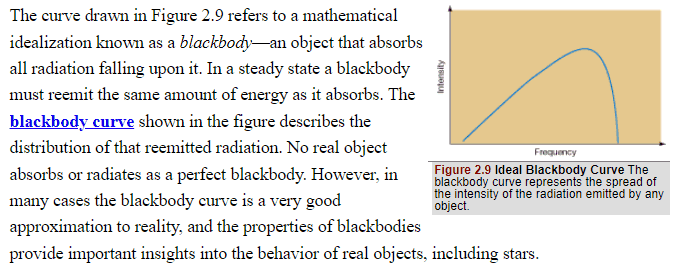This source, https://ase.tufts.edu/cosmos/view_picture.asp?id=1332 , also such a spectrum which doesn't extend toward infinity.

Is it spectrum shown above assuming emissivity of less than 1?

If spectrum of a blackbody peaks at, say, frequency of f=10 kHz at temperature of 400 K. How will such a spectrum relate to a non-ideal blackbody at the same temperature of 400 K? Will the spectrum of the non-ideal blackbody also peak around f=10 kHz but the intensity goes to almost zero for limited range of frequencies around f=10 kHz?

Thank you for the help, in advance!

•256bits
PainterGuy

I thought about it before posting it in classical physics section. Since my questions were not about quantum aspect therefore I decided to post in classical physics section.

Homework Helper
Gold Member
2022 Award
I like the blackbody spectrum shown below. It does not seem to extend toward infinity.

It does, you are just fooled by the axes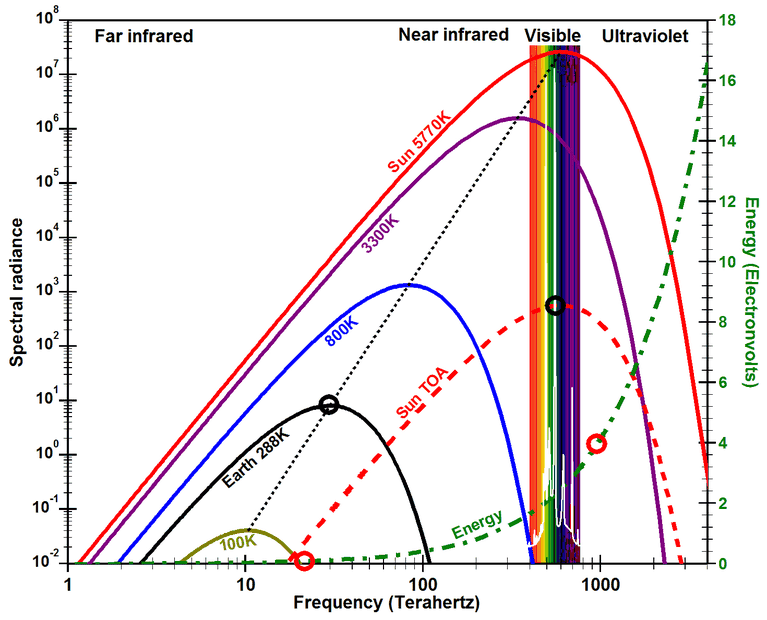Note the logarithmic scales on the axes. You can go down vertically how much you want, there is no limit.

•dextercioby, PainterGuy and Lord Jestocost
Gold Member
•PainterGuy
Gold Member
2022 Award
Planck's law describes the spectral radiance from a black body. As a function of frequency, the spectral radiance ##I(\nu,T)## is given in ‘energy per unit time per unit surface area per unit solid angle per unit frequency’. Occasionally, one writes Planck’s law in terms of energy density, ##u(\nu,T) =\frac {4\pi} c I(\nu,T)##, given in ‘energy per unit volume per unit frequency’.
The derivation is pretty simple, given that the em. field is a bosonic massless spin-1 field:
$$\mathrm{d} U = \frac{2V}{(2 \pi)^3 \hbar^3} \mathrm{d}^3 p \frac{cp}{\exp(\beta c p)-1}.$$
Here ##\vec{p}## are the photon momenta, and the factor 2 takes into account the two polarization degrees of freedom of the photons; ##U## is the energy of the radiation; ##\beta=1/(k_{\text{B}} T)## with ##T## the temperature.

Now instead of ##\vec{p}## we can as well introduce ##\vec{k}=\vec{p}/\hbar## and consider the energy density ##u=U/V##:
$$\mathrm{d} U = \frac{2}{(2 \pi)^3} \mathrm{d}^3 k \frac{\hbar c k}{\exp(\beta \hbar c k)-1}.$$
Finally we can use ##\omega=c k## and integrate over the solid angle
$$\mathrm{d} u= \frac{2}{(2 \pi)^3 c^3} 4 \pi \mathrm{d} \omega \omega^2 \frac{\hbar \omega}{\exp(\beta \hbar \omega)-1}=\mathrm{d} \omega \frac{\hbar \omega^3}{\pi^2 c^3} \frac{1}{\exp(\beta \hbar \omega)-1}.$$

•dextercioby, PainterGuy, Lord Jestocost and 1 other person
Gold Member
Real objects never behave as full-ideal black bodies, and instead the emitted radiation at a given frequency is a fraction of what the ideal emission would be.
I think the problem here is in the definition of a black body and the description of how it behaves. The basic model of a BB starts with a hole in the side of a box which has an internal temperature T. Rayleigh - Jeans started the work but Planck introduced the idea the quantum. Just how big the hole is would be up to the range of wavelengths but the basic theory just assumes it's 'big enough'.

Thinking in terms of antenna theory, the hole would need to be several wavelengths across and of an irregular shape so there would be no resonances or 'frequency sensitive directivity'. For a real object / surface the emissivity would also have to be flat with frequency. The measurements which were used to verify the theory would have referred to optical and IR frequencies and relatively large radiating objects.

I like the blackbody spectrum shown below. It does not seem to extend toward infinity.
That's because of the vertical scale. There are no units marked on the y axis. The formula includes an exponential term and there is no value of x for which ex is zero

•PainterGuy and malawi_glenn
PainterGuy
Thank you, everyone, for the help! Appreciate it.

The following was my reply to @DrClaude in post #6. Could someone please confirm if I had it correct?
I'm glad you asked me about this. I think it has to do with the standing waves in a cavity. I think a metallic cavity of an appropriate size could be used which radiates also radio waves since the surface of metal could act nodes for the standing waves.

If spectrum of a blackbody peaks at, say, frequency of f=10 kHz at temperature of 400 K. How will such a spectrum relate to a non-ideal blackbody at the same temperature of 400 K? Will the spectrum of the non-ideal blackbody also peak around f=10 kHz but the intensity goes to almost zero for limited range of frequencies around f=10 kHz? In other words, is there a quick way to establish a general relation between an ideal blackbody and non-ideal blackbody at the same temperature? Or, the only way is to find the spectrum for an non-ideal blackbody by doing an experiment?

Homework Helper
2022 Award
If spectrum of a blackbody peaks at, say, frequency of f=10 kHz at temperature of 400 K. How will such a spectrum relate to a non-ideal blackbody at the same temperature of 400 K? Will the spectrum of the non-ideal blackbody also peak around f=10 kHz but the intensity goes to almost zero for limited range of frequencies around f=10 kHz? In other words, is there a quick way to establish a general relation between an ideal blackbody and non-ideal blackbody at the same temperature? Or, the only way is to find the spectrum for an non-ideal blackbody by doing an experiment?
Objects radiate exactly as they absorb (this is called the "principle of detailed balance"). To do otherwise would violate thermodynamic law and one could create free work.

So it gets oxymoronic to talk about a non-ideal blackbody because that is usually called "a body"or a "colored object". The black body is an idealization most useful for discussing objects at temperatures far different from than their surroundings and how they radiate

The spectrum of a non-ideal blackbody can be anything you want. Look at the room around you.

•PainterGuy, vanhees71 and Lord Jestocost
Gold Member
... spectrum of the non-ideal blackbody
The simplest case of non-ideal 'blackbody' is called a 'gray body':

Real materials emit energy at a fraction – called the emissivity – of black-body energy levels. By definition, a black body in thermal equilibrium has an emissivity ε = 1. A source with a lower emissivity, independent of frequency, is often referred to as a gray body.

https://en.wikipedia.org/wiki/Black_body

### 10. Radiative heat transfer - EPFL​

•PainterGuy and vanhees71
PainterGuy
Thank you, everyone!

The following quotes are taken from post #18.

The following was my reply to @DrClaude in post #6. Could someone please confirm if I had it correct?
I'm glad you asked me about this. I think it has to do with the standing waves in a cavity. I think a metallic cavity of an appropriate size could be used which radiates also radio waves since the surface of metal could act nodes for the standing waves.

I though to rephrase my above question.

The following, cavity with a hole, is a widely used model for a blackbody. The wavelength of radio waves could be in millimeter and kilometer range. Isn't the cavity size going to affect what kind wavelengths it can support? If the size is too small, how can it support a radio wave with wavelength larger than its size?

Also, the radio waves could penetrate through many materials. Is the material of cavity assumed to be metallic in case of radio waves so that they cannot pass through?

### Cavity with a hole​

A widely used model of a black surface is a small hole in a cavity with walls that are opaque to radiation. Radiation incident on the hole will pass into the cavity, and is very unlikely to be re-emitted if the cavity is large. The hole is not quite a perfect black surface—in particular, if the wavelength of the incident radiation is greater than the diameter of the hole, part will be reflected. Similarly, even in perfect thermal equilibrium, the radiation inside a finite-sized cavity will not have an ideal Planck spectrum for wavelengths comparable to or larger than the size of the cavity.

Suppose the cavity is held at a fixed temperature T and the radiation trapped inside the enclosure is at thermal equilibrium with the enclosure. The hole in the enclosure will allow some radiation to escape. If the hole is small, radiation passing in and out of the hole has negligible effect upon the equilibrium of the radiation inside the cavity. This escaping radiation will approximate black-body radiation that exhibits a distribution in energy characteristic of the temperature T and does not depend upon the properties of the cavity or the hole, at least for wavelengths smaller than the size of the hole.Source: https://en.wikipedia.org/wiki/Black_body#Cavity_with_a_hole

The following section doesn't contain any specific questions but you are welcome to check it for errors. Please add to it if you like to make it more accurate and informative.

I had been thinking about why they were interested in finding how an object radiates at different temperatures. Also, what physical objects did they use in their experiments to check their theoretical laws and how they conducted their experiments? Did they have some kind of almost ideal blackbody? What was the maximum temperature they achieved? How did they vary temperature? How did they heat up the object?

I thought it'd be a good idea to share my own attempt to find the answers.

I think it would have been quite natural for scientists of 19th century to take an interest into the study of blackbody related problems since the field of thermodynamics was in full swing. Most scientists would like to know how an object radiates as its temperature increases. Increasing the efficiency of steam engines could have been another reason.

The figure below shows the comparison of Rayleigh–Jeans law with Wien approximation and Planck's law, for a body of 5800K temperature.Source: https://en.wikipedia.org/wiki/Rayleigh–Jeans_law

Planck's Law is more accurate, then comes Wien's Law or approximation, and the least accurate is Rayleigh-Jeans Law.

I've highlighted important pieces in blue in three quotes below.

In 1900, the British physicist Lord Rayleigh derived the λ^−4 dependence of the Rayleigh–Jeans law based on classical physical arguments and empirical facts. A more complete derivation, which included the proportionality constant, was presented by Rayleigh and Sir James Jeans in 1905. The Rayleigh–Jeans law revealed an important error in physics theory of the time. The law predicted an energy output that diverges towards infinity as wavelength approaches zero (as frequency tends to infinity). Measurements of the spectral emission of actual black bodies revealed that the emission agreed with the Rayleigh–Jeans law at low frequencies but diverged at high frequencies; reaching a maximum and then falling with frequency, so the total energy emitted is finite.
Source: https://en.wikipedia.org/wiki/Rayleigh–Jeans_law#Historical_development

Comparison to Planck's law
In 1900 Max Planck empirically obtained an expression for black-body radiation expressed in terms of wavelength λ = c/ν (Planck's law):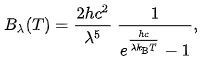where h is the Planck constant and kB the Boltzmann constant. The Planck's law does not suffer from an ultraviolet catastrophe, and agrees well with the experimental data, but its full significance (which ultimately led to quantum theory) was only appreciated several years later.
Source: https://en.wikipedia.org/wiki/Rayleigh–Jeans_law#Comparison_to_Planck's_law

The Planck's law would have taken some time to gain traction since it required complete break from the ideas of classical physics and also Planck himself was in doubt when he came up with his formula.

Wien's approximation (also sometimes called Wien's law or the Wien distribution law) is a law of physics used to describe the spectrum of thermal radiation (frequently called the blackbody function). This law was first derived by Wilhelm Wien in 1896. The equation does accurately describe the short wavelength (high frequency) spectrum of thermal emission from objects, but it fails to accurately fit the experimental data for long wavelengths (low frequency) emission.

Wien derived his law from thermodynamic arguments, several years before Planck introduced the quantization of radiation.

Wien's original paper did not contain the Planck constant.
Source: https://en.wikipedia.org/wiki/Wien_approximation

How they were conducting their experiments, radiators are used, and other details are covered well in the history section of a Wikipedia article on Planck's law, https://en.wikipedia.org/wiki/Planck's_law#History . I will quote a piece, as an image, https://photos.app.goo.gl/uxoU9wqQkmKJUwqM7 , which shows how Planck used the laws of Wien's and Rayleigh's to come up with his own formula.

The quote below is taken from this source, https://chem.libretexts.org/Bookshe...ody_Radiation_Cannot_Be_Explained_Classically . It also has some other interesting information.

By the 1890’s, experimental techniques had improved sufficiently that it was possible to make fairly precise measurements of the energy distribution of blackbody radiation. In 1895, at the University of Berlin, Wien and Lummer punched a small hole in the side of an otherwise completely closed oven, and began to measure the radiation coming out. The beam coming out of the hole was passed through a diffraction grating, which sent the different wavelengths/frequencies in different directions, all towards a screen. A detector was moved up and down along the screen to find how much radiant energy was being emitted in each frequency range. They found a radiation intensity/frequency curve close to the distributions in Figure 1.1.3 .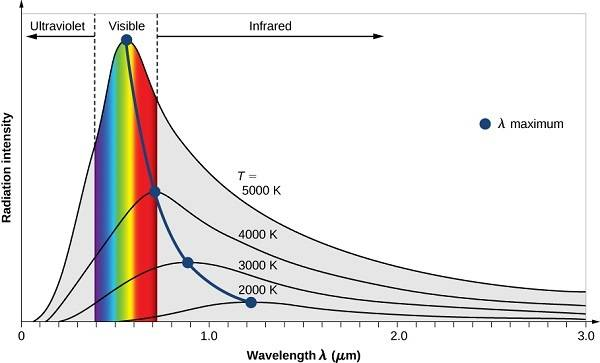Figure 1.1.3 : Graphic representation of spectral distribution of blackbody radiation at different temperatures. The Stefan-Boltzmann’s Law is observed as the increase in the emission amplitude with increasing temperature and the Wien’s Displacement Law is observed as the shift to smaller wavelength with increasing temperature.

By measuring the blackbody emission curves at different temperatures (Figure 1.1.3 ), they were also able to construct two important phenomenological Laws (i.e., formulated from experimental observations, not from basic principles of nature): Stefan-Boltzmann’s Law and Wien’s Displacement Law.

Note to self: If frequency is used on x-axis instead of wavelength, the peak will shift toward right as the temperature increases; check here https://energyeducation.ca/encyclopedia/Blackbody_radiation

Blackbody, Grey body, Real body:
https://photos.app.goo.gl/zEMVSAZRQqb7xSmE9

Homework Helper
2022 Award
The following, cavity with a hole, is a widely used model for a blackbody. The wavelength of radio waves could be in millimeter and kilometer range. Isn't the cavity size going to affect what kind wavelengths it can support? If the size is too small, how can it support a radio wave with wavelength larger than its size?

Also, the radio waves could penetrate through many materials. Is the material of cavity assumed to be metallic in case of radio waves so that they cannot pass through?
It probably can't. But for the Microwave background radiation the cavity is the entire universe so maybe not a concern? Usually you just make it big enough for your system, and the energy at long wavelengths is de minimis
The walls are presumed absorbtive (therefore emissive) and thick enough and at temperature. ("Small"aperture in "infinite" substrate at T)
In reality it is a very useful model

•PainterGuy, Lord Jestocost and vanhees71
Gold Member
The wavelength of radio waves could be in millimeter and kilometer range. Isn't the cavity size going to affect what kind wavelengths it can support?
That follows. But this cavity is just assumed to be 'big enough' to contain several wavelengths of the lowest frequency EM wave that's of interest. Also I think you can assume a cavity 'shape' that would have as many modes as you would want. The fact that the curve tends to zero as the wavelength increases so any error would be less and less relevant.

•PainterGuy
PainterGuy
Thank you!

Question 1:
Doesn't a cavity blackbody source only support a certain wavelengths which are integer multiples of each other? If that's true, the generated spectrum would be discrete at magnifying level.

Question 2:
This question is about the quote given below. The quote implies that before 1898, the exposed incandescent solid body was primarily used as a blackbody source in experiments.

Was that exposed incandescent solid body more like an incandescent light bulb, or just a heated solid spherical metallic ball?

In 1898, Otto Lummer and Ferdinand Kurlbaum published an account of their cavity radiation source. Their design has been used largely unchanged for radiation measurements to the present day. It was a platinum box, divided by diaphragms, with its interior blackened with iron oxide. It was an important ingredient for the progressively improved measurements that led to the discovery of Planck's law. A version described in 1901 had its interior blackened with a mixture of chromium, nickel, and cobalt oxides.

The importance of the Lummer and Kurlbaum cavity radiation source was that it was an experimentally accessible source of black-body radiation, as distinct from radiation from a simply exposed incandescent solid body, which had been the nearest available experimental approximation to black-body radiation over a suitable range of temperatures. The simply exposed incandescent solid bodies, that had been used before, emitted radiation with departures from the black-body spectrum that made it impossible to find the true black-body spectrum from experiments.
Source: https://en.wikipedia.org/wiki/Planck's_law#History

Gold Member
Doesn't a cavity blackbody source only support a certain wavelengths which are integer multiples of each other?
This is sort of true for a one dimensional cavity with a simple set of overtones but, once the cavity is cuboidal, there are many more possible modes, considering all the possible paths you could draw at different angles. The separation between adjacent mode wavelengths will be as close as you like. Make the cavity a complicated irregular polyhedron and the modes become even more densely packed. Then you have to take into account the bandwidth of these modes when the walls are hot. That will give you a continuum of possible wavelengths - the old Engineers' "near enough" comes to mind.

•PainterGuy
Homework Helper
2022 Award
The problem with a glowing orb is mostly the fact that no surface is actually black (meaning uniformly 100% absorbing/emitting across the spectrum) or even gray (meaning uniformly <100% absorbing/emitting across the spectrum). If one uses a cavity, the radiation emitted from the aperture will have interacted strongly (bounced around inside the cavity) even if the walls are somewhat reflective. The energy spectrum of the photons then accurately represents the Temperature of the walls.
Also the walls of the cavity will be at a more rigorously uniform Temp.

The issue of counting the modes is used in all branches of physics, and the discreteness is not a real issue as @sophiecentaur says. In cases of strong pervasive directionality (like conduction electrons in a metal crystal) one must take the structure into account. The "shape of the Fermi surface" is one manifestation

•PainterGuy and Lord Jestocost
Gold Member
Is it really true that a blackbody at 37 C radiates microwaves and radio waves etc. as shown in the figure below? I don't think so. Then, why is the curve shown this way?
Microwave radiation is detected as noise from warm objects, such as the ground. For instance, the receiving antenna on a satellite is looking at a warm Earth, whereas the receiving antenna on Earth is looking at a cold sky. It has a lower "noise temperature" and can therefore detect a weaker wanted signal.
An antenna delivers a noise power to the receiver equal to kTB Watts, where k is Boltzmann's Constant, T is the noise temperature in Kelvin and B is the bandwidth of the system in Hertz.
If the warm object is transparent to microwaves then it does not radiate noise, so a lossless dielectric does not do so and neither does a "perfect" reflector, such as a sheet of metal.

•PainterGuy
PainterGuy
If one uses a cavity, the radiation emitted from the aperture will have interacted strongly (bounced around inside the cavity) even if the walls are somewhat reflective. The energy spectrum of the photons then accurately represents the Temperature of the walls.

Thank you!

I think I get your point. The radiation or photons coming out of orifice in Fig 1 is better representative of the range of photons (i.e. wavelength) emitted at a certain temperature T for a blackbody.

On the other hand, if one analyzes the photons coming out from the circled area of the surface in Fig 2, it would not represent the entire range of photons emitted at temperature T. Also, I think at microscopic scale the temperature might vary across a body.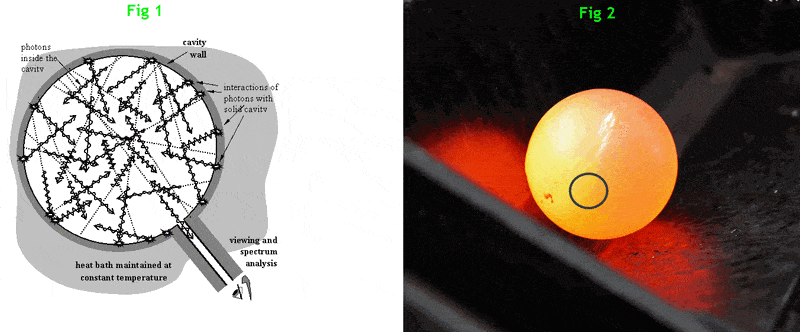By the way, is being "somewhat reflective" a good thing in this scenario?

PainterGuy
Fig 1 shows spectra for a blackbody at different temperatures.

Fig 2 shows relative number of molecules and their speeds at different temperature.

I understand a blackbody is a solid object and the molecules in Fig 2 are gaseous molecules.

Is it okay to make a relation between two figures? In Fig 2 as the temperatures rises, say 1200 K, the curve becomes comparative flattened which means more molecules have speeds close to the average speed. In similar fashion, when temperature rises in Fig 1, say 6000 K, the curve becomes comparatively sharp which means more atoms are radiating the photons with energies close the average peak energy. Could you please guide me with this?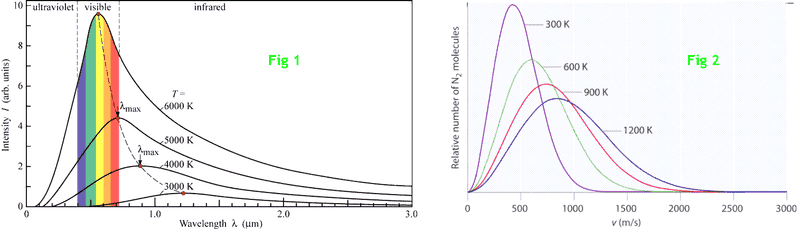Sources for figures:
Fig 2: https://chem.libretexts.org/Bookshelves/General_Chemistry/Map:_Structure_and_Properties_(Tro)/11:_Gases/11.08:_Temperature_and_Molecular__Velocities

••